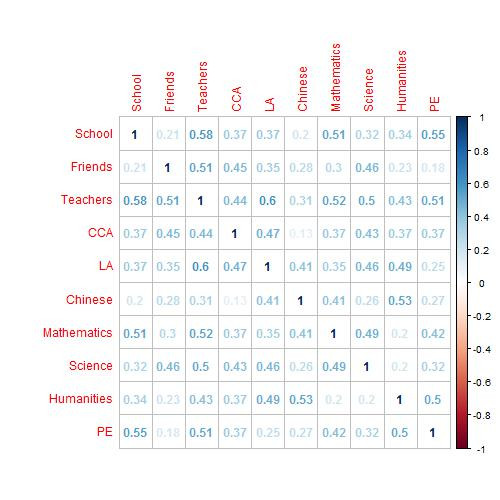Sample size and correlation

A typical question faced is how much data is considered enough. The answer is it depends. First and foremost, we need to know what comprises the total population. If the population is small, and there are enough resources to obtain whatever information you want on the total population, then that is definitely enough - in fact that's the best case scenario. In the event that is not plausible (which is the case for most situations), we conduct a survey. And the next question would be how many people to survey. Again, the answer is it depends. 1. It depends on the size of the total population. 2. It depends on the margin of error you're willing to accept. 3. It depends on the confidence interval (typically 0.95). Also, we have to ensure that when we perform the sample selection, the sample is representative of the population and that they are randomly selected (i.e. everyone has an equal chance of being selected).

There are online calculators that give us the appropriate number of responses to collect for a certain margin of error and confidence level to estimate effect sizes for means and proportions. The larger the sample sizes, the more likely we are able to detect small effect sizes. How about in the case of correlations where we are typically interested in large correlation coefficients (as it means we can try to change one variable to have an impact on another)? Does it mean that the larger the sample size, the more we will be able to detect strong correlations? As a rule of thumb, statisticians consider an r-value of 0.7 to be strong.

Let's take a look at this example. A survey is conducted on two-thirds of the student cohort of a certain level and we observe that there is no strong correlation between the responses to any pair of the questions.Based on the 116 survey responses, we observe that the correlation coefficient ranges from 0.11 to 0.59. Next I want to find out if there are differing results with smaller sample sizes.All survey respondents (N=116)

I then performed bootstrapping and selected random samples of 50 respondents ten times from the total pool of survey respondents. Even though the sample size is now smaller, there are strong correlations observed for bootstrapped sample 6 (school v math, school v humanities, math v science) and sample 10 (school v math). So in the event that we actually only polled the sample of respondents in bootstrapped sample 6 (to represent the whole population), we would have made a conclusion that there is a strong correlation between those variables. And this shows that having a large sample size doesn't mean that we are more likely to observe stronger correlations; where in this case, a larger sample size actually weakens the correlation. This goes to show that what matters more is understanding the homogeneity of the population and how we perform the sampling (i.e. whether the sample is randomly selected and representative of the stratification of the population). A smaller sample with high homogeneity will display a greater correlation coefficient than a large sample with low homogeneity (high heterogeneity). So if we choose to focus on a population that is homogeneous, we might not need a large sample size to reflect the correlation. Of course, if we want to be conservative, we can adjust the threshold of which we consider a strong correlation, while considering the confidence interval of correlation coefficient. There are online calculators and also a package in R that can be used for computing for the confidence interval of the correlation coefficient. It takes into account the observed sample correlation coefficient, sample size and confidence level (typically 0.95).

Here, I would also like to reference a paper on "At what sample size do correlations stabilize?", for which results indicate that in typical scenarios the sample size should approach 250 for stable estimates. This would be a trivial solution in this case as it means I have to poll the entire population (the student cohort is <250). In other words, we should try to obtain a larger sample whenever possible. The comment from Chris Draheim in a thread, "What is the minimum sample size to run Pearson's R?", on ResearchGate also highlights the instability of small samples: "I wouldn’t trust any correlation without at least 50 or 60 observations, with 80-100 being around where I feel comfortable. From my experience with pilot data and analyzing subsets of datasets or presenting data on an ongoing study, correlations with 20-40 subjects can be markedly different than when you have 80-100, I’ve even seen correlations between two tasks going from -.70 to +.40 when the observations were doubled.it’s also important to identify outliers, even with larger sample sizes an outlier or two can have a large effect on the magnitude of the correlation, since this is least squares after all."

Separately, it would be interesting to study how the profile of bootstrapped sample 6 differs from the rest to show such differences in the correlation analysis.Bootstrapped sample 1Bootstrapped sample 2Bootstrapped sample 3Bootstrapped sample 4Bootstrapped sample 5Bootstrapped sample 6Bootstrapped sample 7Bootstrapped sample 8Bootstrapped sample 9Bootstrapped sample 10

References:

https://www.surveysystem.com/correlation.htm

https://www.checkmarket.com/blog/how-to-estimate-your-population-and-survey-sample-size/

https://www.r-bloggers.com/how-to-calculate-confidence-intervals-of-correlations-with-r/

[/Edit] This post has also been published in Towards Data Science on Medium.

Tags:

1,880 views